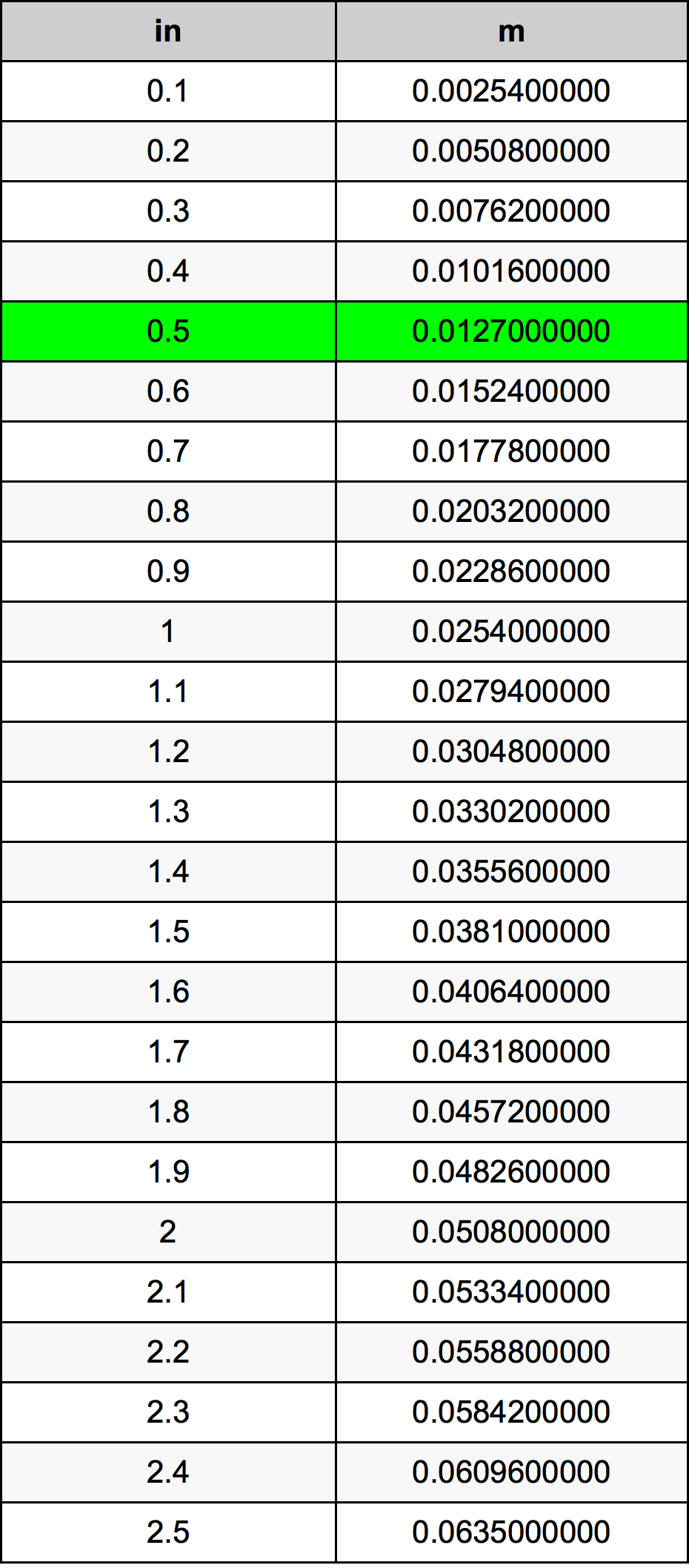Inches To Meters

# 0.5 in to m0.5 Inches to Meters

in
=
m

## How to convert 0.5 inches to meters?

 0.5 in * 0.0254 m = 0.0127 m 1 in
A common question is How many inch in 0.5 meter? And the answer is 19.6850393701 in in 0.5 m. Likewise the question how many meter in 0.5 inch has the answer of 0.0127 m in 0.5 in.

## How much are 0.5 inches in meters?

0.5 inches equal 0.0127 meters (0.5in = 0.0127m). Converting 0.5 in to m is easy. Simply use our calculator above, or apply the formula to change the length 0.5 in to m.

## Convert 0.5 in to common lengths

UnitUnit of length
Nanometer12700000.0 nm
Micrometer12700.0 µm
Millimeter12.7 mm
Centimeter1.27 cm
Inch0.5 in
Foot0.0416666667 ft
Yard0.0138888889 yd
Meter0.0127 m
Kilometer1.27e-05 km
Mile7.8914e-06 mi
Nautical mile6.8575e-06 nmi

## What is 0.5 inches in m?

To convert 0.5 in to m multiply the length in inches by 0.0254. The 0.5 in in m formula is [m] = 0.5 * 0.0254. Thus, for 0.5 inches in meter we get 0.0127 m.

## 0.5 Inch Conversion Table## Alternative spelling

0.5 Inch to Meters, 0.5 Inch in Meters, 0.5 Inches to Meters, 0.5 Inches in Meters, 0.5 in to m, 0.5 in in m, 0.5 in to Meters, 0.5 in in Meters, 0.5 Inch to Meter, 0.5 Inch in Meter, 0.5 Inches to m, 0.5 Inches in m, 0.5 Inch to m, 0.5 Inch in m